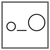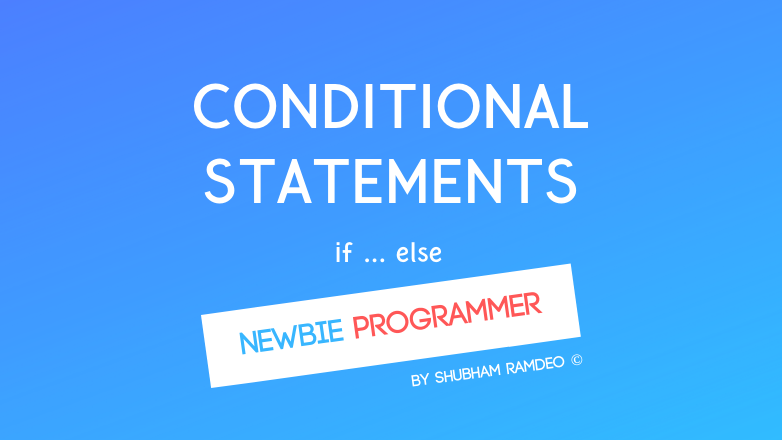Fuddlepixel coz tech is fun();

# conditional statements

Let us discuss how to use conditional statements to make a computer to take right decisions, by logic and if - else statements.

By Shubham Ramdeo
19 September 2015 · 6 mins read
# `c`  `newbie`This is how logic comes to play, Conditional Statements make a computer to take right decisions.

Welcome to the Newbie Programmer Series ! In the last post (click here) we have discussed about flow charts. We are currently learning about Controlling the flow of program and Flow charts makes it easy for us to understand the complex flow of our code. As we have done that, now we can easily understand the coding stuffs. In this post we will learn one of the awesome thing in programming, the Conditional Statements. These are the if else. With these, we can take the decisions. But before that, if you are new to this series, please go to the index (click here) and read out all the previous parts so that you can easily understand what is going down below.

In a recent post : Miscellaneous Operators , I have already discussed the conditional operator. The if and else are just that. I have also prepared some flowcharts to make the things easy to understand. So Lets discuss the power of conditions below. Please continue below.

## The if statement

First see the usage :-

``````...
if ( condition )
{
// code if condition is true
}
...
``````

You just have to give a condition inside if ( .. ). If that condition is found true by the program, the code inside the if’s { and } will be executed.

You can understand the flow using this flow chart :The if flowchart

Now an example will elaborate everything better :

``````#include<stdio.h>
main()
{
int num;
printf("Hello there, Want to give a number ? \n");
scanf ("%d", &num);
if(num == 3)
{
printf("Oh ! that's a magic number ! \n");
}
printf("Thanks ! \n");
}
``````

OUTPUT:

``````Hello there, Want to give a number ?
8
Thanks !

Hello there, Want to give a number ?
3
Oh ! that's a magic number !
Thanks !
``````

You see, whatever number you will enter, you will get thanks only but only when you enter 3, you will get the magic number message. Note that we have used == because we are testing the equality and assigning something so = will not work here. Read more : find the difference between = and ==

Now the thing is that if the condition is true, first the if part will execute than program continues. But If the condition is false then if part will be skipped and the program will continue. But we want some certain action if the condition is false, for that, we need “else”

## The else statement

Lets first see how to use :

``````...
if (condition)
{
//code if condition is true
}
else
{
//code if condition is false
}
...
``````

The “else” part is used when the condition is false. Now you can do the thing like : if condition is true, do the if part then follow rest. if the condition is false, follow the else part then do the rest. Its different from the previous part, see the last para. Or better use the below flow chart.The if else flowchart

Now compare both flow charts above, we will notice the difference easily.

Lets do an example :

``````#include<stdio.h>
main()
{
int num;
printf("Please enter 3 ? \n");
scanf ("%d", &num);
if(num == 3)
{
printf("Oh ! that's a magic number ! \n");
}
else
{
printf("That's not 3 ! \n");
}
printf("Bye ! \n");
}
``````

OUTPUT:

``````Please enter 3 ?
3
Oh ! that's a magic number !
Bye !

Please enter 3 ?
7
That's not 3 !
Bye !
``````

You see the difference, now we have a another piece of code that will run if the condition is not true. But what if we want different outputs for different conditions ? Simple, we can use the nested if else !

## Nested if else

Nested if else is used when we need more than one test expression. Or its like having different outputs for different results on a same test.

lets see the how to use :

``````...
...
if (condition A)
{
//code for condition A true
}
else if (condition B)
{
//code for condition B true
}
else if (condition C)
{
//code for condition C true
}
...
else
{
//code for all conditions false
}
...
...
``````

This is actually like this :

``````...
...
if (condition A)
{
//code for condition A true
}

else

if (condition B)
{
//code for condition B true
}

else

if (condition C)
{
//code for condition C true
}
...
else
{
//code for all conditions false
}
...
...
``````

But I feel the first form is easy to understand. Well both are exactly the same.

This flow chart will help us :The nested if else flow chart

Now again an example but with different conditions :

``````#include<stdio.h>
main()
{
int num;
printf("Please enter 0 or 1 or 2 \n");
scanf ("%d", &num);
if(num == 0)
{
printf("Do you know division by zero is not defined ! \n");
}
else if(num == 1)
{
printf("Do you know 1 is a factor of every number ! \n");
}
else if(num == 2)
{
printf("Do you know its the first even number ! \n");
}
else
{
printf("ERROR : I asked for 0 or 1 or 2 only !!! \n");
}
printf("Bye ! \n");
}
``````

You will find different outputs for 0 and 1 and 2. But if I put something else It will say the last error message. and Bye will by printed every time.

This was a long post isn’t it ? Well practice with your own conditions, and let me finish this for today. We have completed if else and tomorrow, we will learn goto statement. With goto, we can jump in the code ! But Do you know, respectable programmers don’t find using goto a good practice. Why ? for that, you have to read my next post. Stay connected !

##### Update me weekly ✉

Subscribe below and we’ll send you a weekly email summary of all new Code tutorials. Never miss out on learning about the next big thing.

#### Shubham RamdeoShubham Ramdeo is the founder of Fuddlepixel. He is a geek, a writer, involved with many technical and humanity projects.

Meet me!

#### Recent#### Related

`c`

`newbie`

Top